## Sunday, January 24, 2010

### Irodov Problem 2.10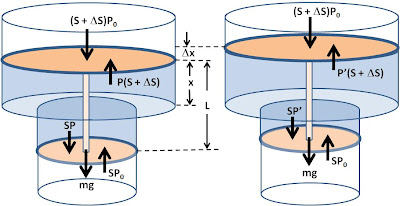Let the surface area of the smaller piston be S then the surface are of the larger piston is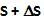. Let the initial pressure due to the gas in the volume between the pistons be P. Let the final pressire between the pistons after heating be P'. Let the distance between the pistons be L and let x be the height of upper piston from the point where the cross section of the chamber changes (as depicted in the pciture).

First let us consider all the forces acting on the two Piston system before it was heated. There are five forces acting on the two piston system. i) the total weight of the two pistons acting downwards mg, ii) the force on the upper piston due to external pressureacting downwards iii) the force on the upper piston due to the volume of gas between the pistonsacting upwards iv) the force on the lower piston due pressure between the two pistons PS acting downwards and v) the force on the lower piston due external pressure PoS acting upwards. Since the two piston system is in equilibrium (not accelerating) we have,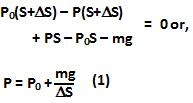The same argument can be used to derive the condition for equilibrium after heating as,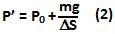Let V be the total volume of gas between the pistons before heating,Let V' be the total volume of gas between the pistons after heating,Let the initial temperature be T and that after heating be.

Since there is 1 mole of gas in the volume between the pistons, from Ideal gas law we have,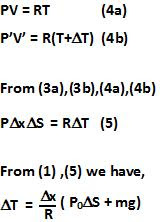1.2.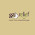3.